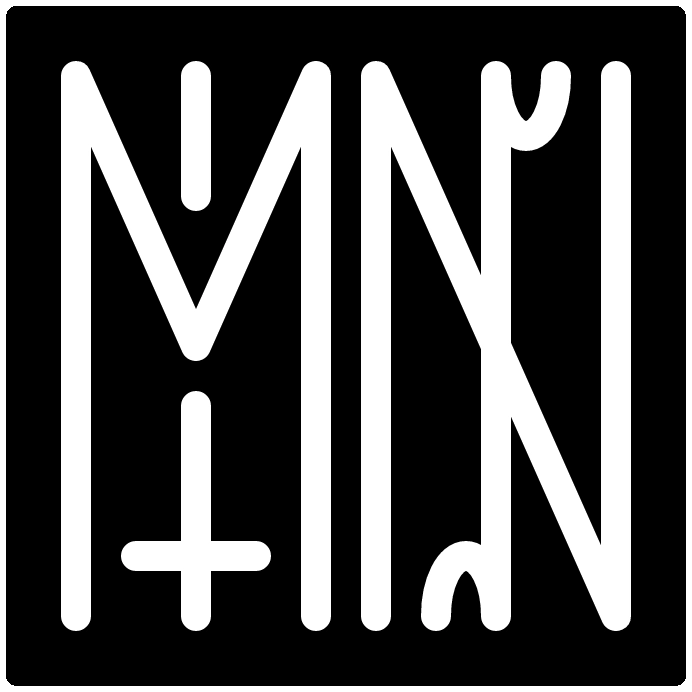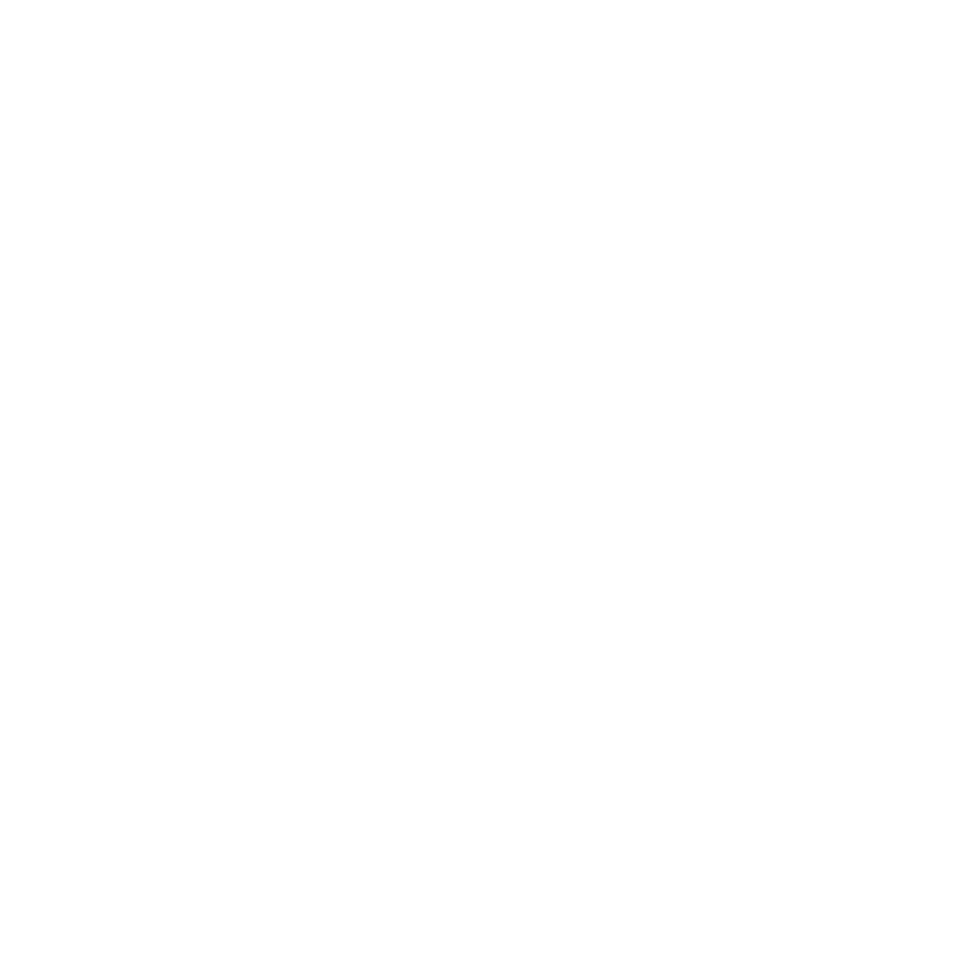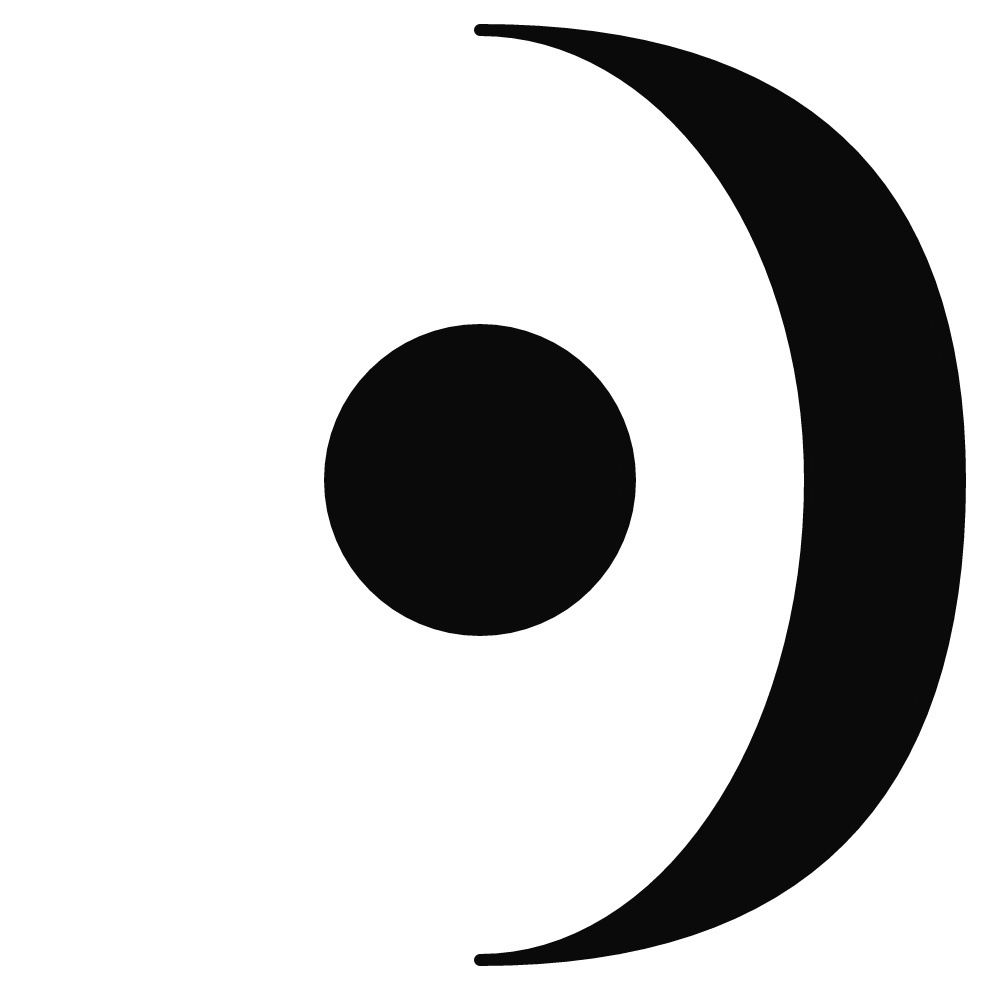directory
projects
linguistics
misc
audio
recordings
visual
temporality
identity# electrical engineering notes

i never studied electrical engineering, and generally am a complete novice. below are some notes i've been taking as i learn. i'm also taking notes on specific [integrated-circuits] while learning about making a [diy-synthesizer].

# laws

## ohms law

I = current (amperes) V = voltage (volts) R = resistance (ohms)

``````I = V / R
V = I * R
R = V / I
``````

## faraday's law

predicts how a magnetic field will interact with an electric circuit to produce a electromagnetic field, which is, electromagnetic induction

The electromotive force around a closed path is equal to the negative of the time rate of change of the magnetic flux enclosed by the path.

# units

• Voltage (volts): electric potential difference
• Ampere (amps) = electrical current
• Ohm (Ω) = electrical resistance
• Farad = capacitance of electrical potential
• K = shorthand for 1000 Ohms often
• also 2K8 means 2800 for example

# components

## diode

one side has no resistance, other is infinite (ideally). they ensure current flows in one direction

cathode - short leg: negative end anode - long leg: positive end

light emitting diode (LED) - converts electricity into light

## resistor

resistors simply resist current! then converts current into heat.

resistors are made out of insulators and conductors. more insulators is equal to more resistance.

resistors have stripes on them. read about the electronic color code.

resistors also can serve as heating elements. if you think about a toaster, or a hair dryer, they function by means of the same mechanism as resistors.

water analogy: smaller pipe = bigger resistor

## capacitors

stores electric charge in an electric field. it is :

electrolitic capacitors are bigger - they are polarized. cermaic capacitors don't store very much charge - not polarized.

water analogy: a bucket that empties when full sorta?

## potentiometer

a three terminal resistor.

there is dial against a graphite disk the more graphite that it has to go through, the more resistance. the pivot point is connected to the middle. side pins are the opposite sides.

the terms are called:

• nose of the potentiometer (middle)
• cheek of the potentiometer (ends)

## integrated circuit

a specific set of capacitors, inductors, transistors, resistors in a circuit.

these look like small "chips". "living bug" position refers to it being with its legs down. and the flat part is at the top.

the pins on an IC have numbers like so:

``````10 9 8 7 6
| | | | |
|----------|
|\         |
| |        |
|/         |
|----------|
| | | | |
1 2 3 4 5
``````

where the left side has a marker like a half circle

## voltage divider

• passive linear circuit that reduces voltage from its input to its output
• one way to create a voltage divider is to use a potentiometer - a resistive divider
• If you set up two resistors - VoltageOut = Resistance2 / (Resistance1 + Resistance2) * VoltageIn

## BJT

• biopolar junction transistor
• uses the language of:
• emitter
• base
• collector

## FET

• field effect transistor
• uses the language of:
• source
• gate
• drain

## VCC VEE VDD VSS

• terms for various voltage supplies on schematics

• Negative Voltage Supplies

• VCC: Voltage Collector Collector
• VDD: Voltage Drain Drain
• Positive Voltage Supplies

• VEE: Voltage Emitter Emitter
• VSS: Voltage Source Source

Read more about the history of these names here.

## op-amp

• op-amp is short for operational amplifier
• it can also be used as a buffer
• if you connect the negative out back to the post gain out it can be negative feedback
• using this buffer, we can add resistance to the feedback loop to control the total voltage added

# specific integrated circuits

i decided to start keeping track of specific ICs in the [integrated-circuits] page.

## CMOS 4000 Series

• there are a lot of CMOS 4000 series ICs to do various things
• check wikipedia for datasheets and descriptions

# schematics

within electrical engineering, we need to read schematics. they're designs that specify the components of a electrical circuit. reading schematics mostly involves learning a number of small icons used as a visual language to represent components. see this useful sparkfun guide on reading schematics.

# bom

BOM usually stands for bill of materials - a list of all the components or eletronics or pieces used in a circuit or build.

linked from: integrated-circuits now
last edited: 2021.05.19